## Info

Publication number
JP2013150117A
JP2013150117A JP2012008229A JP2012008229A JP2013150117A JP 2013150117 A JP2013150117 A JP 2013150117A JP 2012008229 A JP2012008229 A JP 2012008229A JP 2012008229 A JP2012008229 A JP 2012008229A JP 2013150117 A JP2013150117 A JP 2013150117A
Authority
JP
Japan
Prior art keywords
bank
reference voltage
correction
capacitor
voltage
Prior art date
Legal status (The legal status is an assumption and is not a legal conclusion. Google has not performed a legal analysis and makes no representation as to the accuracy of the status listed.)
Pending
Application number
JP2012008229A
Other languages
Japanese (ja)
Inventor
Hirotomo Ishii

Tomohiko Sugimoto

Masanori Furuta

Original Assignee
Toshiba Corp

Priority date (The priority date is an assumption and is not a legal conclusion. Google has not performed a legal analysis and makes no representation as to the accuracy of the date listed.)
Filing date
Publication date
Application filed by Toshiba Corp, 株式会社東芝 filed Critical Toshiba Corp
Priority to JP2012008229A priority Critical patent/JP2013150117A/en
Publication of JP2013150117A publication Critical patent/JP2013150117A/en
Application status is Pending legal-status Critical

• 238000006243 chemical reaction Methods 0 abstract 2
• 230000001276 controlling effects Effects 0 abstract 1
• 230000001603 reducing Effects 0 abstract 1
• 230000001629 suppression Effects 0 abstract 1

## Images

••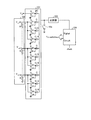•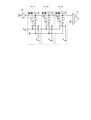•••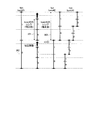••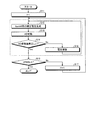•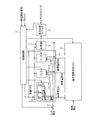•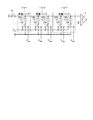•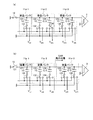••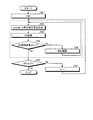••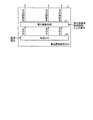•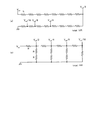•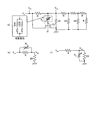•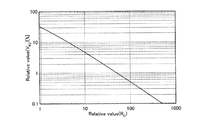••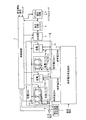•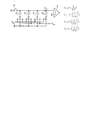•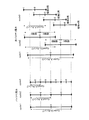•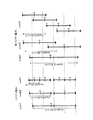•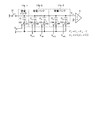••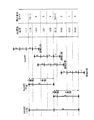••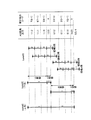••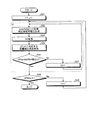••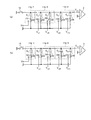•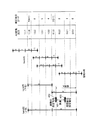•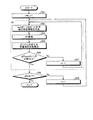•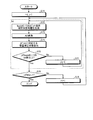••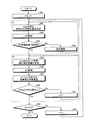•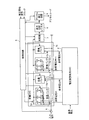••## Classifications

• HELECTRICITY
• H03BASIC ELECTRONIC CIRCUITRY
• H03MCODING; DECODING; CODE CONVERSION IN GENERAL
• H03M1/00Analogue/digital conversion; Digital/analogue conversion
• H03M1/66Digital/analogue converters
• H03M1/68Digital/analogue converters with conversions of different sensitivity, i.e. one conversion relating to the more significant digital bits and another conversion to the less significant bits
• H03M1/687Segmented, i.e. the more significant bit converter being of the unary decoded type and the less significant bit converter being of the binary weighted type
• HELECTRICITY
• H03BASIC ELECTRONIC CIRCUITRY
• H03MCODING; DECODING; CODE CONVERSION IN GENERAL
• H03M1/00Analogue/digital conversion; Digital/analogue conversion
• H03M1/12Analogue/digital converters
• H03M1/34Analogue value compared with reference values
• H03M1/38Analogue value compared with reference values sequentially only, e.g. successive approximation type
• H03M1/46Analogue value compared with reference values sequentially only, e.g. successive approximation type with digital/analogue converter for supplying reference values to converter
• H03M1/466Analogue value compared with reference values sequentially only, e.g. successive approximation type with digital/analogue converter for supplying reference values to converter using switched capacitors
• H03M1/468Analogue value compared with reference values sequentially only, e.g. successive approximation type with digital/analogue converter for supplying reference values to converter using switched capacitors in which the input S/H circuit is merged with the feedback DAC array
• HELECTRICITY
• H03BASIC ELECTRONIC CIRCUITRY
• H03MCODING; DECODING; CODE CONVERSION IN GENERAL
• H03M1/00Analogue/digital conversion; Digital/analogue conversion
• H03M1/66Digital/analogue converters
• H03M1/74Simultaneous conversion
• H03M1/76Simultaneous conversion using switching tree
• H03M1/765Simultaneous conversion using switching tree using a single level of switches which are controlled by unary decoded digital signals
• HELECTRICITY
• H03BASIC ELECTRONIC CIRCUITRY
• H03MCODING; DECODING; CODE CONVERSION IN GENERAL
• H03M1/00Analogue/digital conversion; Digital/analogue conversion
• H03M1/66Digital/analogue converters
• H03M1/74Simultaneous conversion
• H03M1/80Simultaneous conversion using weighted impedances
• H03M1/802Simultaneous conversion using weighted impedances using capacitors, e.g. neuron-mos transistors, charge coupled devices
• H03M1/804Simultaneous conversion using weighted impedances using capacitors, e.g. neuron-mos transistors, charge coupled devices with charge redistribution

## Abstract

PROBLEM TO BE SOLVED: To provide an analog-digital converter that reduces an error in an analog-digital conversion result while suppressing an increase in circuit area.SOLUTION: The analog-digital converter includes: a capacitive DAC having one or more capacitive elements, at least one of which is connected to an input signal, to generate an output signal depending on the connection state of the capacitive elements; a reference voltage generation circuit for supplying reference voltages to the capacitive DAC; a comparator for outputting a comparison result depending on the output signal; a successive approximation register for outputting a digital signal depending on the comparison result from the comparator; and a control circuit for controlling the connection state of the capacitive elements in accordance with the comparison result. The control circuit compares a conversion result that is a digital signal derived from a sample of a predetermined voltage with an ideal code that is an errorless ideal digital signal derived from the sample, and performs corrective control for reducing an error in the digital signal in accordance with the result of comparison.

## Description

The present embodiment relates to an analog-digital converter and a receiver.

In a successive approximation ADC (Analog to Digital Converter), a reference voltage is input to a capacitor DAC (Digital to Analog Converter), the set voltage of the capacitor DAC is compared with the input voltage, and the capacitor DAC is controlled so that they are closest to each other. Thus, the input voltage is converted into a digital code.

The capacitor DAC is composed of a plurality of capacitor banks (capacity arrays), and a threshold is set by a set voltage of each capacitor bank, and bit determination is performed using the threshold. For this reason, there is a problem that if there is a deviation in the set voltage, a deviation occurs in the threshold, and an error occurs in the conversion result (digital code) due to the deviation in the threshold. Factors that cause the set voltage deviation include variations in each capacitor constituting the capacitor DAC, variations in the reference voltage when a plurality of reference voltages are used, and the like.

K. H. Hadidi, V. S. Tso and G. C. Temes, "An 8-b 1.3-MHz Successive-Approximation A / D Converter," JSSC, Jun, 1990. F. Kuttner, "A 1.2V 10b 20MSample / s non-binary successive approximation ADC in 0.13um CMOS," ISSCC 2002, pp. 176-177, 2002. A.N.Karanicolas, H.S.Lee and K.L.Bacrania, "A 15-b 1-MSsample / s Digitally Self-Calibrated Pipeline ADC," JSSC, pp. 1207-1215, Dec 1993.

The present embodiment provides an analog-to-digital converter that can reduce an error in an analog-to-digital conversion result while suppressing an increase in circuit area.

According to one aspect of the present invention, the capacitor DAC includes one or more capacitor elements, at least one of the capacitor elements is connected to an input signal, and generates an output signal in accordance with the connection state of the capacitor elements; A reference voltage generating circuit for supplying the reference voltage to the DAC, a comparator for outputting a comparison result according to the output signal, a successive approximation register for outputting a digital signal according to the comparison result by the comparator, and a comparison by the comparator A control circuit that controls the connection state of the capacitive element according to the result, and the control circuit is an ideal digital signal that has no error when sampled as a conversion result that is a digital signal when a predetermined voltage is sampled. The ideal code which is a signal is compared, and control is performed so as to perform correction for reducing the error of the digital signal in accordance with the comparison result. In addition, the capacitive element connected to the input signal is connected to the reference voltage instead of the input signal. Among the capacitive elements, the connection destination of the capacitive DAC does not change during the comparison and is connected during sampling. A predetermined voltage is determined in accordance with a difference between a reference voltage connected at the time of sampling and a reference voltage connected at the time of comparison in a capacitor having a different reference voltage connected at the time of comparison.

Exemplary embodiments of an analog-digital converter and a receiver will be described below in detail with reference to the accompanying drawings. In addition, this invention is not limited by this embodiment.

(First embodiment)
FIG. 1 is a block diagram illustrating a configuration example of an analog-to-digital converter (hereinafter referred to as an AD converter) according to the first embodiment. The AD converter according to the present embodiment is an analog-digital converter called a successive approximation ADC. As shown in FIG. 1, a capacitor DAC1, a voltage adjustment resistor DAC2, a comparator 3, a successive approximation register 4, and a control circuit 5 are used. , A switch 6 and a correction voltage generation circuit 7 are provided.

The capacitor DAC1 includes a switch 12 and capacitor banks 11-1 to 11-M (M is an integer of 1 or more). Each of the capacity banks 11-1 to 11-M includes N (N is an integer of 1 or more) capacitors (capacitance elements) and N switches. The voltage adjustment resistor DAC2 generates a reference voltage #k (k = 1, 2,... M). The reference voltage #k is supplied from the voltage adjustment resistor DAC2 to the capacitor bank 11-k (k = 1, 2,... M). The comparator 3 outputs a comparison result corresponding to the difference between the sampled voltage and the voltage corresponding to the connection state of the capacitive element in the capacitor DAC1, according to the output signal from the capacitor DAC1. In the present embodiment, the comparison result from the comparator 3 is input to the control circuit 5 via the successive approximation register 4, but the comparison result is directly input from the comparator 3 to the control circuit 5. You may comprise.

Here, the basic operation of the successive approximation type AD converter using a plurality of reference voltages will be described using a configuration example of the successive approximation type AD converter to be compared. FIG. 2 is a diagram illustrating a configuration example of a successive approximation AD converter to be compared. The successive approximation AD converter of the comparative example includes a capacitor DAC 101, a resistor DAC 102, a comparator 103, and a successive approximation ADC control circuit (Digital Circuit) 104.

As a technique for reducing the area of the capacitor DAC, there is a technique in which the capacitor DAC is divided into a plurality of capacitor banks (arrays) and different reference voltages are used in the respective capacitor banks. FIG. 2 shows a configuration example of a successive approximation AD converter using a plurality of reference voltages in this way. In such an AD converter, a reference voltage input to each capacitor bank is weighted based on a voltage (Vref) that determines an input range to the AD converter. The weight of the reference voltage is determined by the resolution of the capacitor array. For example, if there are two capacity banks, upper and lower, and the upper resolution is Na, the upper is Vref and the lower is Vref / 2 Na .

The AD converter shown in FIG. 2 includes one sample capacitor (2C) and three capacitor banks (capacitance array). Each capacity bank is composed of three types of capacitors C, C / 2, and C / 4. Each capacity is, for example, a capacitor. Reference voltages of Vref, Vref / 8, and Vref / 64 are supplied to the respective capacity banks. One end of all capacitors is connected to a common terminal (a terminal for connecting all capacitors to one common signal line), and a signal line connecting the common terminals is connected to an input of the comparator 103.

There are two basic operations of this circuit: (a) signal sample and (b) successive approximation. (B) Successive comparison includes (b-1) MSB (Most Significant Bit) conversion cycle for MSB conversion, (b-2) Conversion cycle for conversion of bits one bit lower than the MSB (MSB-1) conversion cycle, The conversion is sequentially performed for each bit according to the required resolution as shown in FIG.

(A) In the signal sampling, the switch 105, the switch S1, the switches S3, S5, S7, S9, S11, S13, S15, S17, and S19 are turned on (other switches are turned off), and the sample (input voltage Vin) ) Is held. (B) In the successive comparison, the switch S1 is turned off and the switch S2 is turned on, and the states of the other switches differ depending on each conversion cycle.

In the case of the circuit of FIG. 2, in the (b-1) MSB conversion cycle, the switches S4, S5, S7, S9, S11, S13, S15, S17, and S19 are turned on, and based on the comparison result of the comparator 103 in this state. Then, either switch S3 or switch S4 is turned ON (the switch having the smaller difference from the input voltage is turned ON when ON). (B-2) (MSB-1) In the conversion cycle, S6, S7, S9, S11, S13, S15, S17, and S19 are turned ON. At this time, one of the switches S3 and S4 is turned on according to the conversion result of the (b-1) MSB conversion cycle. (B-1) The switch S4 is turned on when the conversion result of the MSB conversion cycle is “1”, and the switch S3 is turned on when the conversion result of the (b-1) MSB conversion cycle is “0”. Thereafter, conversion cycles are sequentially performed for each bit up to LSB. With this operation, the voltage Vout input to the comparator 103 is expressed by the following equation (1).

Here, Vref2 indicates the reference voltage of the second capacity bank, and Vref3 indicates the reference voltage of the third capacity bank. The reference voltage of the first capacitor bank is Vref, which is the same voltage that determines the input range of the AD converter. D  to D  indicate digital codes (that is, digital signals) obtained by the comparator 103. As shown in FIG. 2, since each reference voltage is Vref2 = Vref / 8 and Vref3 = Vref / 64, the above equation (1) becomes the following equation (2).

If the resistor DAC 102, which is a reference voltage generation circuit, can generate binary weighted voltages that are exactly 1/8 and 1/64, respectively, as Vref2 and Vref3 by Expression (2), accurate AD conversion can be performed. Is possible. However, if the binary weights for Vref2 and Vref3 are not accurate, an error occurs in the AD conversion of the second capacity bank and the third capacity bank, which causes a miscode. As described above, when a plurality of reference voltages are used, variations in the reference voltage cause an error in the conversion result.

FIG. 3 is a diagram illustrating a configuration example of the capacitor DAC1 of the present embodiment. In FIG. 3, the number of capacity banks is three (capacity banks 11-1 to 11-3), and each capacity bank has one capacity (capacitance value 1C) for simplicity. Specifically, the capacity bank 11-1 includes a capacity (capacitance element) 121 and a switch 131, the capacity bank 11-2 includes a capacity 122 and a switch 132, and the capacity bank 11-3 includes a capacity 123 and a switch. 133 is provided. Therefore, each capacity bank corresponds to 1 bit, and in the configuration example of FIG. 3, 3-bit AD conversion can be performed.

2 has a sample capacity for holding the input voltage Vin (sample), but FIG. 3 does not include a sample capacity, and each capacity also has a function as a sample hold. Show. Each capacitor can be connected to Vin. (A) In the signal sample, the switch 12 is turned ON, and the switches 131, 132, and 133 are connected to Vin.

The operation when the successive approximation AD conversion method of the comparative example is implemented in the configuration example of FIG. 3 will be described. First, the basic operation of the successive approximation type AD converter of the comparative example in the case where the reference voltage is not varied and is ideal will be described. FIG. 4 is a diagram illustrating the concept of successive approximation AD conversion of the comparative example. FIG. 4 shows the concept of successive approximation AD conversion based on the configuration of the capacity bank shown in FIG. The vertical axis in FIG. 4 represents voltage, and the horizontal axis represents time ((b) conversion cycle of each bit by successive comparison). The reference voltage Vref is supplied to the capacitor bank 11-1, the reference voltage Vref2 = Vref / 2 is supplied to the capacitor bank 11-2, and the reference voltage Vref3 = Vref / 4 is supplied to the capacitor bank 11-3. Supplied. In the following description of the present embodiment, the capacity bank 11-k (k = 1, 2, 3) is abbreviated as bank # k.

FIG. 4 shows the input ranges and threshold voltages of the banks # 1, # 2, and # 3 (that is, the capacity banks 11-1, 11-2, and 11-3). The threshold voltage indicates the voltage at the boundary between the output code of each bit being “0” or “1”. The input range 201 indicates the input range of bank # 1, the input range 202 indicates the input range of bank # 2, and the input range 203 indicates the input range of bank # 3. The threshold voltage 204 indicates the threshold voltage of bank # 1, the threshold voltages 205 and 206 indicate the threshold voltage of bank # 2, and the threshold voltage 207 indicates the threshold voltage of bank # 3.

In the successive approximation type AD conversion method of the comparative example, in (b) successive approximation, the conversion cycle of each bit is executed in order from the MSB (corresponding to bank # 1). The input range of each capacity bank is the reference voltage of each bank. Therefore, the input range 201 of bank # 1 is Vref, the input range 202 of bank # 2 is Vref / 2, and the input range 203 of bank # 3 is Vref / 4. If the reference voltage can be set as ideal, there is no fogging (overlap) between the input ranges and no gap (missing) between the ranges, and AD conversion is performed accurately.

Specifically, for example, when the conversion result in bank # 1 is “1”, the input range 202 of bank # 2 corresponds to the upper half above the threshold voltage 204 that is the center of the input range 201 of bank # 1. To do. When the conversion result in bank # 1 is “0”, the input range 206 of bank # 2 corresponds to the lower half of the threshold voltage 204 that is the center of the input range 201 of bank # 1. Then, the threshold voltage 205 of the bank # 2 when the conversion result in the bank # 1 is “1” is the center of the input range 202. That is, the threshold voltage 205 of bank # 2 when the conversion result in bank # 1 is “1” is a position ¼ from the top of the input range 201 of bank # 1. Similarly, the threshold voltage 206 of bank # 2 when the conversion result in bank # 1 is “0” is a position ¼ from the bottom of the input range 201 of bank # 1.

There is no particular limitation on the normal AD conversion operation in the AD converter of this embodiment, that is, the AD conversion operation for the input voltage (external voltage) to be converted, and the same method as in the comparative example can be used. Specifically, the control circuit 5 controls the switch 6 to connect the input voltage and the capacitor DAC1. Then, the control circuit 5 controls each switch in the capacitor DAC 1 to perform (a) signal sample and (b) successive comparison. (B) In successive approximation, in each conversion cycle, a capacitor corresponding to a bit to be converted is connected to a reference voltage. The output from the capacitor DAC 1 is input to the comparator 3, and the bit value corresponding to the successive approximation register 4 is set according to the comparison result of the comparator 3. When the conversion cycle for all bits is completed, the value set in the successive approximation register 4 is output as an AD conversion result (digital code (digital signal)). In addition, although a fog (overrange) between the input ranges may be intentionally provided, even in that case, the conversion from the higher-order bits sequentially is the same as in the comparative example.

FIG. 5 is a diagram illustrating a concept of successive approximation AD conversion in a comparative example when an error occurs in the reference voltage of bank # 2. In the example of FIG. 5, it is assumed that the reference voltage of bank # 2 becomes smaller than the ideal value. The input range 201 is the same as the example of FIG. The threshold voltages 205 and 206 indicate the threshold voltage of bank # 2 in the ideal case shown in FIG. Input ranges 211 and 212 show the actual input range of bank # 2 when an error occurs in the reference voltage of bank # 2, and input ranges 215 to 218 show an error in the reference voltage of bank # 2. The actual input range of bank # 3 is shown. The threshold voltages 213 and 214 indicate the actual threshold voltage of bank # 2 when an error occurs in the reference voltage of bank # 2, and the threshold voltages 219 to 222 are errors in the reference voltage of bank # 2. The actual threshold voltage of bank # 3 in the case where the error occurs is shown.

As shown in FIG. 5, the threshold voltages 213 and 214 are lower than the ideal threshold voltages 205 and 206, and this difference causes a miscode. Specifically, when a reference voltage error as shown in FIG. 5 occurs, an overlap occurs in the actual input range of bank # 3, and the output code (ADC Output Code) of the AD converter shown at the right end of FIG. ), “1” and “5” disappear, resulting in a miscode.

The AD converter according to the present embodiment has a function of correcting the reference voltage by the control circuit 5 controlling the voltage adjustment resistor DAC2 in order to prevent generation of a miscode when a reference voltage error occurs.

FIG. 6 is a diagram illustrating the concept of reference voltage correction according to the present embodiment. The example of FIG. 6 shows a state of correction when the reference voltage of bank # 2 is smaller than the ideal value in the configuration example of FIG. An input range 301 indicates the input range of bank # 2 before correction. In such a case, the reference voltage of bank # 2 is corrected in this embodiment. Specifically, the control circuit 5 controls the switch 6 to connect the correction voltage generation circuit 7 and the capacitor DAC1, and the correction voltage generation circuit 7 inputs a known correction voltage to the capacitor DAC1. The control circuit 5 corrects the reference voltage based on the conversion result of bits equal to or less than the bit corresponding to the capacity bank to be corrected.

In the example of FIG. 6, an example is shown in which the correction voltage 302 that is Vref / 4 is input to the capacitor DAC1. In the example of FIG. 6, when the correction voltage 302 is input, “0” is output in the conversion of bank # 1 (first bit) if it is an ideal reference voltage, and bank # 2 (second bit). “0” should be output in the conversion, and “1” should be output in the conversion of bank # 3 (third bit). In bank # 2, Vref / 4 is an ideal threshold voltage, but here, when Vref / 4 is input, “0” is output, and when Vref / 4 is exceeded, “0” is output. Assume that 1 ″ is output.

On the other hand, as shown in FIG. 6, when the reference voltage of bank # 2 is smaller than the ideal value and there is an error, the threshold voltage is also lowered due to the input range 301 becoming smaller. Therefore, when the reference voltage of bank # 2 has the error shown in FIG. 6, when the correction voltage 302 is input, “1” is output in the conversion of bank # 2, and “1” is output in the conversion of bank # 3. 0 "is output.

In the present embodiment, the control circuit 5 controls the voltage adjustment resistor DAC2 to adjust the reference voltage supplied to the correction target bank based on the conversion result of the bit corresponding to the correction target bank. When bank # 2 is to be corrected, the conversion result of the second and third bits is referred to. In the example of FIG. 6, the conversion result “10” is obtained before correction. The control circuit 5 holds a correct conversion result (hereinafter referred to as an ideal code) corresponding to the value of the correction voltage, and inputs a reference target bit (in this example, “01”) of the ideal code and the correction voltage. The obtained conversion results are compared, and if the ideal code does not match the conversion result, the reference voltage is adjusted. In the example of FIG. 6, since the ideal code and the conversion result do not match, the voltage adjustment resistor DAC2 is controlled to boost the reference voltage supplied to bank # 2, and the boost is stopped when the conversion result becomes “01”. Let Thereby, the reference voltage supplied to bank # 2 can be set to a correct value. The voltage adjustment for boosting is performed digitally, for example, and the voltage adjustment can be accurately performed by setting the adjustment step sufficiently smaller than 1LSB of bank # 1.

Note that even if the ideal code matches the conversion result, the reference voltage is not always correct. For example, when the reference voltage of bank # 2 is larger than the ideal value, “01” may be output as a result of inputting the correction voltage 302 of Vref / 4. Therefore, when the correction voltage 302 of Vref / 4 is input and the values of the second bit and the third bit match the ideal code, it is necessary to check whether the reference voltage of bank # 2 is larger than the ideal value. . As this method, for example, the reference voltage is lowered and the reference voltage is adjusted so that the conversion result becomes a boundary where “10” is reached.

The value of the correction voltage is not limited to Vref / 4, and may be any known value as long as it can be determined whether or not there is an error in the conversion result by inputting to the capacitor DAC1. Further, a plurality of correction voltages may be generated, and the reference voltage may be adjusted based on a plurality of conversion results when each correction voltage is input. For example, it is determined whether the reference voltage is large or small by sequentially inputting two types of upper and lower correction voltages across the threshold voltage and using the conversion results when the respective correction voltages are input. Thereafter, a correction voltage that matches the threshold voltage may be input, and fine adjustment to increase or decrease the reference voltage as described above may be performed. Further, the correction voltage may be different for each bit to be corrected.

As described above, in the reference voltage correction according to the present embodiment, the correction is performed by performing the AD conversion operation using a capacity bank or lower that uses the reference voltage to be corrected. Therefore, the correction process assumes that the lower-order bits are correct reference voltages than the bits to be corrected. From this, for example, correction processing can be performed recursively from the least significant bit to the upper bit in order.

FIG. 7 is a flowchart illustrating an example of a reference voltage correction procedure according to the present embodiment when correction processing is performed from the lowest capacity bank. First, a variable i indicating a capacity bank to be corrected is set to i = M (step S1). As shown in FIG. 1, the capacitor DAC1 includes M capacitor banks of capacitor banks 11-1 to 11-M (bank # 1 to bank #M), and the capacitor bank 11-1 (bank # 1) is an MSB. Correspondingly, it is assumed that the capacity bank 11-M (bank #M) corresponds to LSB (Least Significant Bit).

The control circuit 5 controls to input a correction voltage to the capacitor DAC1 (generates a correction voltage for bank # i) (step S2), and corresponds to bits below bank # i (bits below bit corresponding to bank # i). bank) is used to perform AD conversion (step S3). Then, the control circuit 5 determines whether or not the AD conversion result is correct (step S4).

If the AD conversion result is correct (step S4 Yes), the control circuit 5 determines whether i is 1 or less (step S5). If i is 1 or less (step S5 Yes), the correction process is performed. finish. If i is not 1 or less (No in step S5), i = i-1 is set (step S7), and the process returns to step S2. If the AD conversion result is not correct (No in step S4), the control circuit 5 adjusts the reference voltage (step S6) and returns to step S2.

In step S4, in addition to determining whether the AD conversion result matches the ideal code, as described above, the threshold voltage when the AD conversion result matches the ideal code becomes an ideal position. It also includes confirmation of whether or not That is, when the AD conversion result matches the ideal code, it includes checking whether or not the reference voltage does not match the ideal code if the reference voltage is stepped down (or boosted) even a little. When the reference voltage is stepped down (or boosted) and does not immediately match the ideal code, it can be confirmed that the threshold voltage is in the correct position, and when it does not immediately match the ideal code, the threshold voltage is in the correct position. Therefore, the process proceeds to No in step S4, proceeds to voltage adjustment (step S6), and continues the step-down (or step-up) of the reference voltage until the boundary that does not match the ideal code.

Although the example in which correction processing is performed from the lowest capacity bank has been described above, the order of correction processing is not limited to this. For example, the correction process may be performed from the uppermost capacity bank, or the correction process may be performed from an intermediate uppermost capacity bank.

FIG. 8 is a flowchart showing an example of a reference voltage correction procedure according to the present embodiment in the case where correction processing is performed from the highest capacity bank. First, a variable i indicating the capacity bank to be corrected is set to i = 1 (step S11). Steps S12 to S14 are the same as steps S2 to S4 in FIG. After step S14, the control circuit 5 determines whether i is greater than or equal to M (step S15). If i is greater than or equal to M (step S15 Yes), the correction process is terminated. If i is not equal to or greater than M (No in step S15), i = i + 1 is set (step S17), and the process returns to step S12.

In general, errors in some lower bits including the least significant bit are sufficiently small if they affect the overall performance of the AD converter, and may not cause performance degradation. In this case, the correction process may be performed by removing some lower bits from the correction target.

After the correction processing described above, the control circuit 5 controls the switch 6 to connect the input voltage and the capacitor DAC1, and outputs a correct AD conversion result if normal AD conversion is performed on the input voltage. Can do.

Also, an arbitrary reference voltage may be used instead of the ground voltage shown in FIG. It is sufficient that a residual signal between the input voltage and an arbitrary reference voltage is obtained as the output of the capacitor DAC1. For example, when the reference voltage A is used instead of the ground voltage, if Vref + A is used as the reference voltage, the differential voltage from the reference signal becomes Vref, and the same operation as described above can be realized.

In addition, when a differential circuit is used, the same operation can be realized without a ground voltage by connecting (short-circuiting) terminals corresponding to the ground voltage (ground voltage) of each circuit. From the above, the AD converter according to the present embodiment does not necessarily require a ground voltage.

In this embodiment, the voltage adjustment resistor DAC2 is used as the reference voltage generation circuit that supplies the reference voltage. However, the reference voltage generation circuit only needs to be able to adjust the voltage of the reference voltage to be generated. A reference voltage generation circuit other than the resistor DAC may be used. Similarly in this case, the control circuit 5 may control the reference voltage generated by the reference voltage generation circuit.

As described above, in the present embodiment, the correction voltage is input to the capacitor DAC1, and the control circuit 5 adjusts the reference voltage based on the AD conversion result and the ideal code corresponding to the correction voltage. For this reason, even when a plurality of reference voltages are used, a shift in threshold voltage can be corrected due to variations in reference voltages, and errors in AD conversion results can be reduced.

(Second Embodiment)
FIG. 9 is a block diagram illustrating a configuration example of an AD converter according to the second embodiment. The AD converter of this embodiment is the same as that of the first embodiment except that the correction voltage generation circuit 7 is deleted from the AD converter of the first embodiment and a capacitor DAC1a is provided instead of the capacitor DAC1. is there. Constituent elements having the same functions as those of the first embodiment are denoted by the same reference numerals as those of the first embodiment, and redundant description is omitted.

FIG. 10 is a diagram illustrating a configuration example of the capacitor DAC 1a according to the present embodiment. In the configuration example shown in FIG. 10, the number of capacitors is increased compared to the configuration example in FIG. 3, and a 2-bit AD conversion is possible except for the capacitor bank 11 a-1. In the AD converter of FIG. 10, since the capacity bank 11a-2 covers the output range of the capacity bank 11a-1 by 1 bit, in addition to the input range necessary for normal successive approximation AD conversion, Input range is added). Similarly, the capacity bank 11a-3 is overranged by one bit. For this reason, the reference voltage of the capacity bank 11a-1 is set to Vref, the reference voltage of the capacity bank 11a-2 is set to Vref2 = Vref, and the reference voltage of the capacity bank 11a-3 is set to Vref3 = Vref / 2.

The 1-bit capacity bank 11a-1 includes one 1C capacity (capacitance 121) and a switch 131, and the 2-bit capacity bank 11a-2 includes two capacity 1C and C / 2 (capacities 122 and 124). ) And switches 132 and 134, and the 2-bit capacity bank 11a-3 is composed of two capacitors (capacities 123 and 125) of 1C and C / 2 and switches 133 and 135.

Here, both of the capacity banks 11a-2 and 11a-3 are overranged as 2 bits, but at least one of the capacity banks corresponding to bits below the bit corresponding to the capacity bank to be corrected. One capacity bank should be overranged.

FIG. 11 is a diagram illustrating a circuit state during correction according to the present embodiment. The AD converter according to the present embodiment operates in two modes: a mode in which signal sampling is performed (signal sample mode) and a mode in which successive approximation is performed (successive comparison mode). In the example of FIG. 11, the reference voltage Vref2 of the capacity bank 11a-2 is the correction target. FIG. 11A shows a circuit state in the signal sample mode, and FIG. 11B shows a circuit state in the successive approximation mode.

As shown in FIG. 11A, in the signal sample mode, the switch 12 is turned on, the capacitor 124 of the capacitor bank 11a-2 is connected to Vref2 by the switch 134, and the other capacitors are connected to the ground voltage. As shown in FIG. 11B, in the successive approximation mode, the switch 12 is turned OFF, and the capacitor 122 of the capacitor bank 11a-2 to be corrected and the capacitor 121 of the capacitor bank 11a-1 corresponding to the upper bit are grounded. The AD conversion is performed using the capacitor 124 and the capacitor bank 11a-3 of the capacitor bank 11a-2 to be corrected. In the successive approximation mode, conversion is not started from the MSB, but AD conversion is started from a bit lower than the capacitor bank using the reference voltage to be corrected.

FIG. 12 is a diagram illustrating the concept of reference voltage correction according to the present embodiment. In the following description of the present embodiment, the capacity banks 11a-k (k = 1, 2, 3) are abbreviated as “bank # k”. The voltage set by the circuit in FIG. 12 is a voltage obtained by the signal sampling mode of FIG. 11A, and ideally, it is at a position ¼ from the bottom of the input range Vref of bank # 1. Corresponding voltage 304.

On the other hand, when the voltage set in the circuit before correction is smaller than the ideal value, as indicated by the voltage 303 before correction, the AD conversion output by bank # 3 indicates “1”. When the reference voltage Vref2 is boosted from this state, the AD conversion output by bank # 3 eventually becomes “2”. Since the ideal code in this case is “2”, the voltage adjustment stops in a state where “2” is reached. Assuming that the reference voltage of bank # 3 is ideally Vref / 2, the input range of bank # 2 in the state of “2” matches the ideal value.

In this way, the output code of the AD converter is expanded by overrange (so that not only “1” but also “2” can be expressed), so that the comparison with the ideal value uses the reference voltage to be corrected. This can be implemented using AD conversion results of bits lower than the bank. This means that unlike the first embodiment, it is not necessary to perform AD conversion using a capacitor array that uses a reference voltage to be corrected. Further, in the present embodiment, the correction voltage generation circuit 7 is not provided, but a reference voltage corresponding to the correction voltage is generated using a reference voltage to be corrected and a capacitor bank using the reference voltage and used as a sample signal. Yes. That is, among the capacitive elements, the connection destination does not change during the comparison, and the reference voltage connected at the time of comparison is different from the reference voltage connected at the time of comparison with the reference voltage connected at the time of comparison. A voltage corresponding to the correction voltage is determined according to the difference from the connected reference voltage.

The voltage adjustment is performed digitally, and the voltage adjustment can be accurately performed by setting the adjustment step to be sufficiently smaller than 1LSB of bank # 3. Further, even if the AD conversion output by bank # 3 becomes “2” before correction, the reference voltage of bank # 2 may be larger than the ideal value. In this case, the AD conversion output is “2”. The reference voltage is stepped down until it changes from 1 to “1”.

The normal AD conversion operation (AD conversion operation other than the correction operation) in the AD converter of this embodiment is not particularly limited, and for example, a method similar to that of the comparative example can be used.

In the present embodiment as well, as in the first embodiment, correction processing is performed on the assumption that the lower bits are the correct reference voltage, and therefore correction processing is performed recursively in order from the lowest capacity bank to the upper bits. Can do. FIG. 13 is a flowchart illustrating an example of a reference voltage correction procedure according to the present embodiment when correction processing is performed from the lowest capacity bank. First, the variable i indicating the correction target capacity bank is set to i = M (step S21). As shown in FIG. 9, the capacity DAC 1a includes M capacity banks of capacity banks 11a-1 to 11a-M (bank # 1 to bank #M), and the capacity bank 11a-1 (bank # 1) is an MSB. Correspondingly, it is assumed that the capacity bank 11a-M (bank #M) corresponds to the LSB.

In the sampling signal mode, the control circuit 5 sets the reference voltage supplied to the bank #i as a correction target, and controls to sample the reference voltage supplied to the bank #i (step S22). Next, AD conversion is performed using a bank corresponding to a bit equal to or less than that of bank # (i-1) (step S23).

Then, the control circuit 5 determines whether or not the AD conversion result is correct (step S24). In step S24, in addition to determining whether the AD conversion result matches the ideal code before correction, the threshold voltage is ideal when the AD conversion result matches the ideal code before correction as described above. It also includes confirmation of the correct position.

If the AD conversion result is correct (step S24 Yes), the control circuit 5 determines whether i is 2 or less (step S25). If i is 2 or less (step S25 Yes), the correction process is performed. finish. If i is not 2 or less (No in step S25), i = i-1 is set (step S27), and the process returns to step S22. If the AD conversion result is not correct (No in step S24), the control circuit 5 adjusts the reference voltage (step S26) and returns to step S22.

Although the example in which correction processing is performed from the lowest capacity bank has been described above, the order of correction processing is not limited to this. For example, correction processing may be performed from the most significant capacity bank, or correction processing may be performed from the middle most significant bit.

FIG. 14 is a flowchart illustrating an example of a reference voltage correction procedure according to the present embodiment when correction processing is performed from the highest-level capacitor bank. First, a variable i indicating a capacity bank to be corrected is set to i = 2 (step S31). In the sampling signal mode, the control circuit 5 sets the reference voltage supplied to the bank #i as a correction target, and controls to sample the reference voltage supplied to the bank #i (step S32). Next, AD conversion is performed using a bank corresponding to a bit equal to or less than that of bank # (i + 1) (step S33). Step S34 is the same as step S24 of FIG. After step S34, the control circuit 5 determines whether i is greater than or equal to M (step S35). If i is greater than or equal to M (step S35 Yes), the correction process is terminated. If i is not equal to or greater than M (No in step S35), i = i + 1 is set (step S37), and the process returns to step S32.

As described above, in this embodiment, at least one of the capacity banks corresponding to the bits lower than the capacity bank to be corrected is overranged, and the reference voltage to be corrected is used as the sampling signal without using the correction voltage. The reference voltage is corrected based on the AD conversion result of the bits lower than the correction target bit. For this reason, it is possible to correct the shift of the threshold voltage due to the variation in the reference voltage without generating a correction voltage, and to reduce the error of the AD conversion result.

In the above description, an example of adjusting the reference voltage supplied to the bank to be corrected has been described. It is the same as adjusting the relative relationship. Therefore, the reference voltage supplied to the bank that has been the correction target in the above example may not be adjusted, and the reference voltage supplied to the lower bank may be adjusted. From the viewpoint of adjusting the relative relationship, the same effect as the above example can be obtained. However, the direction in which the reference voltage is increased or decreased is opposite to that in the above example. That is, when the voltage is boosted in the above example, the voltage is reduced. When the voltage is boosted in the above example, the voltage is boosted and adjusted.

(Third embodiment)
FIG. 15 is a diagram illustrating a configuration example of the voltage adjustment resistor DAC2 according to the third embodiment. In the present embodiment, a specific configuration example of the voltage adjustment resistor DAC2 of the AD converter in the first embodiment or the second embodiment will be described. As shown in FIG. 15, the voltage adjustment resistor DAC2 of the present embodiment includes a voltage adjustment circuit 21 and a resistor DAC22. The resistor DAC 22 may have any configuration as long as it is a resistor DAC that outputs divided voltages # 1 to #M with respect to the reference voltage. Based on the control from the control circuit 5, the voltage adjustment circuit 21 adjusts the divided voltages # 1 to #M output from the resistor DAC 22 and outputs the resulting voltages as reference voltages # 1 to #M.

FIG. 16 is a diagram illustrating a configuration example of the resistor DAC 22. There are generally two types of configuration methods for the resistor DAC circuit. One is a method of connecting resistance elements having a resistance value R (resistance element R) in series connection (FIG. 16A), and the other is a method. This is a method of configuring in a series-parallel arrangement of resistors (FIG. 16B) (referred to as R-2R type). When configured using the basic resistance element R, the series connection type requires 16 resistance elements R, and the R-2R type can be configured by 11 resistance elements R. The difference in the number of resistors required for configuring a circuit tends to widen as the resolution improves. For this reason, when configuring a resistor DAC that requires a relatively high resolution, the R-2R type can reduce the number of elements compared to the series connection type and can reduce the area (the number of resistors is 3 bits). Is the same in the two ways, R-2R is more advantageous with 4 bits).

FIG. 17 is a diagram illustrating a circuit configuration example of the voltage adjustment resistor DAC2 using the R-2R type resistor DAC22. 17A shows a circuit configuration example of the R-2R type resistor DAC 22 and the voltage adjustment circuit 21, and FIGS. 17B and 17C show the circuit configuration example shown in FIG. 17A. The example of the circuit state which expanded a part of is shown. As shown in FIG. 17A, the voltage adjustment circuit 21 includes a variable resistor Rc and a step-up / step-down changeover switch Sc.

V R in FIG. 17 corresponds to Vref in FIG. In the example of this circuit, it is assumed that the voltage of V R2 is adjusted. When the voltage adjustment is performed, the step-up / step-down changeover switch Sc connects one end of the variable resistor Rc to either the ground or V R1 node. Specifically, when the reference voltage V R2 is boosted, it is connected to the ground (FIG. 17C), and when it is lowered, it is connected to the V R1 node (FIG. 17B). The variable resistor Rc includes a plurality of resistance values (at least one or more) sufficiently larger than the resistance value R and a plurality of switches. Although FIG. 17A shows a configuration example of a variable resistor, the configuration of the variable resistor is not limited to this.

FIG. 18 is a diagram illustrating an example of the relationship between the resistance value of the variable resistor Rc and the reference voltage V R2 . This figure shows the result of calculation assuming that the voltage is boosted. The horizontal axis indicates the resistance value of the variable resistor Rc (relative value with respect to R), and the vertical axis indicates the voltage value of V R2 (relative value with respect to ideal V R2 = Vref / 2). Thus, the value of V R2 can be adjusted by the resistance value of the variable resistor.

As described above, the R-2R type can reduce the number of elements compared to the series connection type. For example, in the case of 10 bits, the series connection type generally requires 2 10 = 1024 resistors, and the circuit area is large. By adopting the R-2R type configuration, the number of resistors becomes about 3 × 10 = 30, and can be reduced to about 1/30. In the R-2R type, the voltage adjustment function can be realized only by adding a variable resistor in the resistor DAC2, so that the circuit area can be reduced.

FIG. 19 is a diagram illustrating a configuration example of the voltage adjustment resistor DAC2 in which the resistor DAC has a voltage adjustment function. In this manner, a switch is provided for each resistor, and a reference voltage can be generated arbitrarily (with a resolution corresponding to the resistance value of each resistor) by controlling the switch. The control circuit 5 controls the switch of the voltage adjustment resistor DAC2 so as to increase or decrease the reference voltage.

As described above, in the present embodiment, the circuit configuration example of the voltage adjustment resistor DAC2 when the resistor DAC22 is used is shown. As described above, the AD converter circuit of the first embodiment or the AD converter circuit of the second embodiment can be realized by using the voltage adjustment resistor DAC2 thus configured.

(Fourth embodiment)
Next, a voltage correction method according to the fourth embodiment will be described. The configuration of the AD converter circuit of this embodiment is the same as that of the first embodiment or the second embodiment.

In the reference voltage correction of the first embodiment and the second embodiment, there is an AD conversion result and a digital code (ideal code) prepared for each reference voltage, and the comparison result of these two values is obtained. In response, the voltage regulator circuit is controlled. In the present embodiment, the control circuit 5 receives the AD conversion result and the ideal code and inputs a digital comparator that outputs a binary control signal of “0” or “1” depending on whether or not they match. An up / down counter that receives a binary control signal of the digital comparator as input and increments or decrements a counter value of a plurality of bits corresponding to the number of bits of the AD converter circuit output according to the value of the control signal. The control circuit 5 controls the step-up or step-down of each reference voltage by inputting the counter value of the up / down counter to the voltage adjustment resistor DAC2.

As described above, by controlling the voltage adjustment using the up / down counter, the voltage adjustment can be controlled with a simple circuit, and the circuit area can be reduced.

(Fifth embodiment)
FIG. 20 is a diagram illustrating a configuration example of the AD conversion circuit according to the fifth embodiment. The AD converter circuit according to the present embodiment includes the reference voltage generation circuit 8 instead of the voltage adjustment resistor DAC2, the capacitor DAC1b instead of the capacitor DAC1, and the addition of the digital correction circuit 9, according to the first embodiment. It is the same as that of the AD converter circuit. The capacitor DAC1b includes a switch 12 and capacitor banks 11b-1 to 11b-M. Constituent elements having the same functions as those of the first embodiment are denoted by the same reference numerals as those of the first embodiment, and redundant description is omitted.

In the successive approximation AD converter, the capacity is usually weighted in binary. On the other hand, a method of weighting capacity using non-binary weights (hereinafter referred to as non-binary weights) has also been proposed. In this method, it is possible to correct the capacity variation in the digital domain using the redundancy obtained by using a non-binary value.

FIG. 21 is a diagram illustrating a configuration example of the capacitive DAC using the non-binary weights of the comparative example. In this example, the reference voltage is single, and an example of 3 bits (the actual information amount is log 2 (1.8 3 )) is shown for simplicity. The circuit configuration is the same as that of a normal successive approximation AD converter, but the capacitance weight is not binary. The weight of the capacitor C1 used in the successive approximation of the MSB is 4 × (0.9) −1 , and the weight of the capacitor C2 used in the bit one bit lower than the MSB is 2 × (0.9) −2 . , The capacity C3 used in the bits 2 bits lower than the MSB is 1 × (0.9) −3 , and the capacity C4 used in the LSB is 1 × (0.9) −3 . The operation of this circuit is the same as that of a normal successive approximation AD converter. The output Vout of this circuit is expressed by the following equation (3). D  to D  indicate digital codes (that is, AD conversion results) obtained by the comparator. Indicates the Vref reference voltage.

FIG. 22 is a diagram illustrating the concept of the successive approximation operation using non-binary weights and the input range of each cycle in the comparative example of FIG. The signal samples are the same as for binary weights. In the successive approximation operation, cycle 1 is the MSB conversion cycle, cycle 2 is the next (lower) bit conversion cycle, and cycle 3 is the LSB conversion cycle. In FIG. 22, for comparison, the input range corresponding to each cycle in the case of binary weights is shown on the right side, and the input range corresponding to each cycle in the case of non-binary weights is shown on the left side.

In the case of the non-binary weights shown on the left side of FIG. 22, the analog output that is in the region A in cycle 2 is AD converted using the lowest input range (for region A) in cycle 3. Similarly, analog signals in the regions B, C, and D of cycle 2 are AD-converted using the input ranges for region B, region C, and region D of cycle 3, respectively.

As can be seen from FIG. 22, in the binary weight AD conversion, there is no fogging (overlap) between the input ranges, whereas in the non-binary weight AD conversion, there is an overlap.

FIG. 23 is a diagram illustrating the concept of the successive approximation operation using non-binary weights and the input range of each cycle when there is variation in capacity. 23 assumes a case where the capacitor DAC having the configuration example shown in FIG. 21 is used, and assumes that an error due to variation occurs in the capacitor C1. When an error occurs in the capacitance, Vout is expressed by the following equation (4). Note that α is a capacity error due to variation.

α has a positive error and a negative error. Here, the description will be made assuming that a positive error occurs. In this case, as shown in FIG. 23, the threshold voltage of cycle 1 is shifted upward from the ideal value. In the case of binary weight AD conversion, a dead zone occurs due to a shift in threshold voltage, which causes a miscode. On the other hand, in the case of non-binary weight AD conversion, it can be seen that no dead band occurs even if the threshold value is shifted due to the overrange of the lower bits. The ability to perform AD conversion without missing information means that the influence of threshold variation can be corrected in the digital domain. For example, if an ideal input signal is given and AD conversion is performed, it is clear from the above equation (4) that the difference between the ideal result and the actual result becomes an error due to variation.

Thus, in the non-binary weight method, it is possible to correct the capacity variation within a range not exceeding the overrange of the lower bits. In the non-binary weighting method, since the radix is 2 or less, the amount of information is smaller than that of the radix 2 as described above. For this reason, an extra AD conversion cycle is required depending on the setting of the radix.

However, in the non-binary weighting method of this comparative example, since the redundancy is controlled by the capacity weight, the capacity value becomes large. The larger the redundancy, the greater the capacity value. In addition, since the radix is not 2, radix conversion must be performed in the digital domain, and hardware overhead is large.

In this embodiment, unlike the non-binary weighting method of the comparative example, it can be configured with a capacity of a power of 2 (binary weight) despite having redundancy. Redundancy is provided by making the value of the reference voltage provided to each array larger than usual. In other words, the binary DAC successive approximation AD converter can be used as it is for the circuit configuration of the capacitor DAC 1b, and AD conversion can be performed without loss of information even if there is an error due to variations in the capacitance only by adjusting the reference voltage supplied to the capacitor DAC 1b. Ensure redundancy. The error of the AD conversion result can be digitally corrected using the AD conversion result.

FIG. 24 is a diagram illustrating a configuration example of the capacitor DAC 1b according to the present embodiment. In order to simplify the explanation, a 4-bit circuit is used in the example of FIG. In the example of FIG. 24, the capacity bank 11b-1 includes a capacity 141 (capacitance value C 1 ) and a switch 151, and the capacity bank 11b-2 includes a capacity 142 (capacitance value C 2 ) and a capacity 143 (capacitance value C 3 ). The capacitor bank 11b-3 includes a capacitor 144 (capacitance value C 4 ), a capacitor 145 (capacitance value C 5 ), and switches 154 and 155. C 1 = C 2 = C 4 = C and C 3 = C 5 = C / 2. The reference voltage Vref1 is input to the capacitor bank 11b-2, and the reference voltage Vref2 is input to the capacitor bank 11b-3. Note that FIG. 24 shows a state where the switch 6 is set so that the input signal Vin is input to the capacitor DAC 1b, and the switch 6 is omitted.

FIG. 25 is a diagram illustrating an example of a circuit state in the correction operation of the capacitor DAC 1b according to the present embodiment. FIG. 25 is based on the circuit configuration of FIG. FIG. 25A shows the circuit state in the signal sample mode, and FIG. 25B shows the circuit state in the successive approximation operation mode (MSB conversion cycle). In the correction operation, similarly to the first embodiment, the control circuit 5 sets the switch 6 to connect the correction voltage generation circuit 7 and the capacitor DAC 1b.

The correction voltage generation circuit 7 generates a known correction voltage. As shown in FIG. 25A, in the signal sample mode, the capacitor bank 11b-1 samples a signal (in this case, a correction voltage) to which the capacitor 141 (capacitance value C 1 ) is input. Accordingly, one end of the capacitor 141 (capacitance value C 1 ) is connected to the correction voltage generation circuit 7 and one end of each of the capacitors 142 to 145 is grounded. The capacitive DAC output signal line (Vout in FIG. 25) is also grounded.

In the successive approximation operation of FIG. 25B, one end of C 1 is grounded, one end of C 2 is connected to the reference voltage Vref1, and Vout is in a floating state. Vout at this time is expressed by the following equation (5). 24 and 25, the capacitor 142 of the capacitor bank 11b-2 corresponds to the MSB, the capacitor 143 of the capacitor bank 11b-2 corresponds to the bit one bit lower than the MSB, and the capacitor bank 11b-3 The capacity 144 corresponds to the bit that is two bits lower than the MSB, and the capacity 145 of the capacity bank 11b-3 corresponds to the LSB.

The circuit of FIG. 24 performs the successive approximation operation of FIG. 25B up to the LSB cycle for each bit to obtain a 4-bit AD conversion result. When the AD conversion result in each cycle is D  to D , Vout is expressed by the following equation (6).

Here, when Vref1 = Vref / 2 and Vref2 = Vref / 8, the above equation (6) becomes the following equation (7). This equation (7) means that a binary successive approximation operation can be realized by the circuit of FIG.

In this embodiment, in order to perform digital correction, the capacity bank 11b-2 is designed to give an overrange. The overrange gives an amount corresponding to 1 LSB of the capacity bank 11b-2. This can be realized by using Vref / 4 as the reference voltage Vref2 of the capacitor back 11b-3.

26, 27, and 28 are diagrams showing an operation conceptual diagram of the capacitor DAC 1b according to the present embodiment. In the following description of the present embodiment, the capacity bank 11b-k (k = 1, 2, 3) is abbreviated as bank # k. In the example of FIG. 26, it is assumed that a positive error occurs in the threshold voltage in the capacitor (capacitor 143) for determining the lower bits of bank # 2 due to variations in the capacitance and the like. FIG. 27 shows an operation concept of the capacitor DAC1b of the present embodiment when there is no error. In the example of FIG. 28, it is assumed that an error in the negative direction occurs in the threshold voltage in the capacitor (capacitor 143) for determining the lower bits of bank # 2.

In the example of FIG. 26, it is assumed that an error due to a variation in the capacitance occurs in the capacitor (capacitor 143) for determining the lower bits of bank # 2. Here, an error α that can be expressed by C 3 = (C / 2) × (1 + α) is assumed. In this case, the above equation (7) becomes the following equation (8).

Equation (8) means that the threshold voltage of the lower bits of bank # 2 deviates from the ideal value. FIG. 26 shows an operation example when this deviation is 1.5 × (Vref / 8). As shown in FIG. 26, this error causes the threshold voltage to shift upward.

When there is a shift in the threshold voltage, the AD conversion output is as shown at the right end of FIG. Here, the notation of the AD conversion output (a, b, c) in FIG. 26 is the AD conversion output of the upper bits of a: bank # 2, the AD conversion output of the lower bits of b: bank # 2, and c: bank #. 3 AD conversion output (for 2 bits). As digital value weights, 2 2 is used for the AD conversion output of the upper bits of bank # 2, 2 1 is used for the AD conversion output of the lower bits of bank # 2, and 2 0 is used for the AD conversion output of bank # 3. It takes. In this example, there is a problem that a digital code of (0, 1, 1) is lost as an AD conversion output due to a shift in threshold voltage. In this embodiment, the AD conversion output in an error state shown in FIG. 26 is digitally corrected.

In the digital correction of the present embodiment, the control circuit 5 obtains the difference between the AD conversion output and the ideal code as a correction value and passes the correction value to the digital correction circuit 9. The digital correction circuit 9 stores the correction value, and performs digital correction using the correction value in normal AD conversion for the input voltage. For example, assume that Vref1 / 4 is input as the correction voltage as shown in FIG. In this example, since the threshold voltage of the lower bits of bank # 2 is shifted upward, the correction value is + 1LSB. Therefore, if the determination value of the lower bits of bank # 2 is “1”, the correction value “+1” is added to the AD conversion result when the AD conversion output of the lower bits of bank # 2 is “1”. To make corrections. The corrected code is shown at the right end of FIG. Thus, it can be seen that AD conversion can be performed without error codes by adding the correction value “+1” to the AD converter output in accordance with the AD conversion result of the lower bit of bank # 2 to be corrected.

As shown in FIG. 28, when there is an error of −1.5 × (Vref / 8) in the threshold voltage, there arises a problem that a digital code of (0, 0, 1) is lost as an AD conversion output. In the case of this example, when the AD conversion output of the lower bits of bank # 2 is “1”, the correction value “−1” is added to the AD conversion output (that is, 1 is subtracted) to perform correction. . This correction is performed by the digital correction circuit 9.

For example, when Vref1 / 4 ± Δ (Δ is a voltage sufficiently smaller than 1LSB of bank # 3) is used as the correction voltage, if there is no deviation of the threshold voltage, the case of Vref1 / 4 + Δ (0, 1, 0) is output as the AD conversion result, and (0, 0, 1) in the case of Vref1 / 4−Δ is output as the AD conversion result. If ideal values are obtained as AD conversion results for both inputs of Vref1 / 4 ± Δ, there is no need for correction. For at least one of the inputs of Vref1 / 4 ± Δ, a correction value is obtained when an ideal value differs from the AD conversion result.

As shown in FIG. 26, when the threshold voltage of the lower bits of bank # 2 is shifted to the plus side, the case of Vref1 / 4 + Δ (0, 0, 2) is the case of Vref1 / 4−Δ (0, 0, 1). Is output. Conversely, when the threshold value is shifted to the minus side, (0, 1, 1) is output in the case of Vref1 / 4 + Δ, and (0, 1, 1) is output in the case of Vref1 / 4−Δ.

Therefore, if both the low-order bit AD conversion results of bank # 2 at the time of Vref1 / 4 + Δ input and Vref1 / 4−Δ input are 0, it can be assumed that the threshold voltage is shifted in the positive direction, and the correction value is “( (AD conversion result of bank # 3 at the time of (Vref1 / 4 + Δ) input) −1 ”. Further, if the lower bit AD conversion results of bank # 2 at the time of Vref1 / 4 + Δ input and Vref1 / 4−Δ input are both 1, it can be assumed that the threshold voltage is shifted in the negative direction, and the correction value is “0”. − ((AD conversion result of bank # 3 when (Vref1 / 4−Δ) is input) ”.

In this example, the correction value is obtained using the relationship between bank # 2 and bank # 3, but if the overrange is performed with the bank corresponding to one bit among the bits lower than the correction target bit, the same value is obtained. An arbitrary bank correction value can be obtained by the method. Note that the correction value appropriate for obtaining the correction value differs depending on the bank to be corrected, so that the correction voltage generation circuit generates a voltage corresponding to the bank bit to be corrected.

The AD converter of this embodiment has two operation modes: (1) normal operation mode and (2) correction value measurement operation mode. In the normal mode, the control circuit 5 controls the switch 6 to input the input voltage to the capacitor DAC 1b. The digital correction circuit 9 performs digital correction using the correction value obtained in the correction value measurement operation mode. The timing for executing the correction value measurement operation mode may be arbitrary. For example, the correction value is stored when the AD converter is powered on, and the correction value is stored until the next correction value measurement operation mode is executed. Sometimes, digital correction is performed using the stored correction values.

Note that the AD conversion result of the AD converter of this embodiment is a binary code system, and the correction value is also a binary code system. Thus, it is not necessary to perform radix conversion as in the non-binary weight method as in the comparative example, and digital correction can be realized only by addition and subtraction, so that the hardware can be configured easily.

FIG. 29 is a diagram illustrating an example of a correction value calculation procedure according to the present embodiment when a correction value is obtained from the lowest capacity bank. As shown in FIG. 29, first, the control circuit 5 sets i = M and j = 1 (step S41). The position of the capacity indicated by j in the bank #i is defined as the order of correction from the upper bit to the lower bit if the first bit in the bank #i is defined as the most significant bit. If the eye is defined as the least significant in bank # i, the correction value calculation order is from the least significant bit to the most significant bit.

The control circuit 5 controls the correction voltage for the jth bit of bank # i to be input to the capacitor DAC1b (step S42), and performs AD conversion (step S43). Then, a correction value for the capacity corresponding to the j-th bit of bank # i is calculated (step S44).

Next, the control circuit 5 determines whether j is greater than or equal to the number of bits of bank # i (step S45). If j is greater than or equal to the number of bits of bank # i (step S45 Yes), i is It is determined whether it is 1 or less (step S46). If i is 1 or less (step S46 Yes), the correction value calculation process is terminated. If j is not equal to or greater than the number of bits of bank # i in step S45 (No in step S45), j = j + 1 is set (step S47), and the process returns to step S42. In step S46, if i is not 1 or less (No in step S46), i = i-1 is set (step S48), and the process returns to step S42.

FIG. 30 is a diagram illustrating an example of a correction value calculation procedure according to the present embodiment in the case where a correction value is obtained from the highest capacity bank. As shown in FIG. 30, first, the control circuit 5 sets i = 1 and j = 1 (step S51). Control is performed so that the correction voltage for the jth bit of bank # i is input to the capacitor DAC1b (step S52), and AD conversion is performed (step S53). Then, a correction value for the capacity corresponding to the j-th bit of bank # i is calculated (step S54).

Next, the control circuit 5 determines whether j is greater than or equal to the number of bits of bank # i (step S55), and when j is greater than or equal to the number of bits of bank # i (step S55 Yes). I is greater than or equal to M (step S56). If i is equal to or greater than M (Yes in step S56), the correction value calculation process ends. If j is not equal to or greater than the number of bits of bank # i in step S55 (No in step S55), j = j + 1 is set (step S57), and the process returns to step S52. If i is not equal to or greater than M in step S56 (No in step S56), i = i + 1 is set (step S58), and the process returns to step S52.

As described in the first embodiment, the error of some lower bits including the least significant bit has a sufficiently small influence on the overall performance of the AD converter, and therefore correction is performed on some lower bits. You may make it not. Further, an arbitrary reference voltage may be used instead of the ground voltage shown in FIG. In addition, when a differential circuit is used, the same operation can be realized without a ground voltage by connecting (short-circuiting) terminals corresponding to the ground voltage (ground voltage) of each circuit.

In this embodiment, the AD conversion result is corrected by digital correction. However, instead of performing digital correction, a correction voltage is used in the same manner as the reference voltage correction of the first embodiment. The capacitance value itself of the capacitance may be adjusted based on the AD conversion result.

As described above, in this embodiment, the capacitor DAC is configured with binary weights, and the input voltage overlap is provided by adjusting the reference voltage, thereby providing redundancy. Then, a correction value for performing digital correction is obtained based on the AD conversion result when a known correction voltage is input, and digital correction using this correction value is performed in normal AD conversion. For this reason, it is possible to reduce the error of the AD conversion result due to the shift of the threshold voltage while configuring the capacitor DAC with binary weights, and it is not necessary to change the weight of the capacitor, so that the circuit area of the capacitor DAC is reduced. it can.

(Sixth embodiment)
FIG. 31 is a diagram illustrating a configuration example of an AD conversion circuit according to the sixth embodiment. The AD converter of this embodiment is the same as that of the fifth embodiment except that the correction voltage generation circuit 7 is deleted from the AD converter of the fifth embodiment. Components having the same functions as those in the fifth embodiment are denoted by the same reference numerals as those in the fifth embodiment, and redundant description is omitted.

In the present embodiment, a voltage for measuring a correction value, which is a substitute for the correction voltage, is generated by the capacitor DAC 1b itself.

FIG. 32 is a diagram illustrating an operation example of calculating the correction value of the capacitor DAC 1b according to the present embodiment. In FIG. 32, the configuration shown in FIG. 24 of the fifth embodiment is assumed as a circuit configuration example of the capacitor DAC 1b.

FIG. 32A shows an example of the circuit state in the sample mode when obtaining the correction value, and FIG. 32B shows an example of the circuit state in the hold operation mode. In this example, the correction value of the capacitor 143 is obtained with the capacitor 143 (C 3 ) as a correction target. In FIG. 32A, one ends of capacitors 141, 142, 144, and 145 are grounded. A reference voltage Vref1 (= Vref / 2) is input to the capacitor 143.

In the hold operation mode of FIG. 32B, one ends of the capacitors 141 to 145 are grounded, the switch 12 is opened, and the node connected to the comparator 3 is set in a floating state. Vout at this time is expressed by the following equation (9).

Equation (9) means that the output voltage in the hold operation mode is exactly the threshold voltage of the lower bits of bank # 2. Note that α is an error of the capacitor 143 due to a variation in capacitance.

FIG. 33 is a diagram showing an operation concept of the present embodiment. FIG. 33 shows an actual set voltage 402 when there is an ideal set voltage 403 and an error 401 using the output voltage in the hold operation as the set voltage. In the following description of the present embodiment, the capacity bank 11b-k (k = 1, 2, 3) is abbreviated as bank # k. In the measurement of the correction value corresponding to each capacitor of the capacitor DAC1b according to the present embodiment, the voltage set in the hold operation mode is AD-converted using the bank # 3, and is obtained by the difference between the conversion result and the ideal value. Can do.

When outputting the AD conversion result, the output result of the lower bits of bank # 2 is set to “0”. In this example, the actual position of the set voltage 402 exceeds the input range for the area A of bank # 3. In such a case, the voltage is higher than the uppermost threshold voltage and is determined to be “2”. Suppose that Therefore, the AD conversion result in this case is (0, 0, 2). In this example, the ideal value (ideal code) is (0, 0, 1), and the error is 1 LSB from the difference between the actual AD conversion result and the ideal value. Therefore, as in the fifth embodiment, when the lower bit of bank # 2 is output as “1”, correction can be made to an ideal output code by using +1 as the correction value.

When the threshold voltage of the lower bits of bank # 2 is shifted by 1 LSB to the minus side, the AD conversion result is (0, 0, 0). The difference between the actual AD conversion result and the ideal value is −1LSB, which is a correction value. Digital correction operations other than those described above are the same as those in the fifth embodiment.

FIG. 34 is a diagram illustrating an example of a correction value calculation procedure according to the present embodiment when a correction value is obtained from the least significant bit. As shown in FIG. 34, first, the control circuit 5 sets i = M and j = 1 (step S61).

Next, the control circuit 5 controls the correction voltage (setting voltage) for the j-th bit of bank # (i−1) to be input to the capacitor DAC1b (step S62), and performs AD conversion (step S63). ). Then, a correction value for the capacity corresponding to the j-th bit of bank # (i−1) is calculated (step S64).

Next, the control circuit 5 determines whether j is greater than or equal to the number of bits of bank # (i−1) (step S65). If j is greater than or equal to the number of bits of bank # i (step S65 Yes). ), It is determined whether i is 2 or less (step S66). When i is 2 or less (step S66 Yes), the correction value calculation process is terminated. If j is not greater than or equal to the number of bits of bank # i in step S65 (No in step S65), j = j + 1 is set (step S67), and the process returns to step S62. In step S66, if i is not 2 or less (No in step S66), i = i-1 is set (step S68), and the process returns to step S62.

FIG. 35 is a diagram illustrating an example of a correction value calculation procedure according to the present embodiment when a correction value is obtained from the most significant bit. As shown in FIG. 35, first, the control circuit 5 sets i = 2 and j = 1 (step S71). Control is performed so that the correction voltage (setting voltage) for the j-th bit of bank # (i−1) is input to the capacitor DAC 1b (step S72), and AD conversion is performed (step S73). Then, a correction value for the capacity corresponding to the j-th bit of bank # (i−1) is calculated (step S74).

Next, the control circuit 5 determines whether j is greater than or equal to the number of bits of bank # i (step S75). If j is greater than or equal to the number of bits of bank # i (step S75 Yes), i is It is determined whether or not it is greater than or equal to M (step S76). If i is equal to or greater than M (step S76 Yes), the correction value calculation process is terminated. If j is not equal to or greater than the number of bits of bank ## (i−1) in step S75 (No in step S75), j = j + 1 is set (step S77), and the process returns to step S72. If i is not equal to or greater than M in step S76 (No in step S76), i = i + 1 is set (step S78), and the process returns to step S72.

Thus, in this embodiment, since it is not necessary to generate a special signal for measuring the correction value, the circuit area can be reduced as compared with the fifth embodiment.

(Seventh embodiment)
FIG. 36 is a diagram illustrating a configuration example of the AD conversion circuit according to the seventh embodiment. The AD converter of the present embodiment is the same as that of the fifth embodiment except that it includes the voltage adjustment resistor DAC2 of the first embodiment instead of the reference voltage generation circuit 8 of the AD converter of the fifth embodiment. It is the same as the form. Components having the same functions as those of the fifth embodiment and the first embodiment are denoted by the same reference numerals as those of the fifth embodiment and the first embodiment, and redundant description is omitted. .

In the AD converter of this embodiment, a plurality of reference voltages are used. As described in the first embodiment, when variations occur in the plurality of reference voltages, an AD converter error is caused. A method for correcting an error caused by variations in the reference voltage is the same as that in the first embodiment.

In the present embodiment, both the correction of the error due to the variation in the reference voltage and the correction of the error due to the variation in the capacitance similar to the fifth embodiment are performed. FIG. 37 is a diagram illustrating an example of a correction processing procedure according to the present embodiment. There are roughly two correction processes in this embodiment. One is resistance DAC correction (a part surrounded by a square described in FIG. 37A) for correcting an error due to variations in reference voltage, and the other one. One is capacitance DAC correction (a portion surrounded by a square described as FIG. 37B) for obtaining a correction value of an error due to variation in capacitance.

As shown in FIG. 37, first, the control circuit 5 sets i = M and j = 1 (step S81). It is assumed that the capacity bank 11b-1 (bank # 1) corresponds to the MSB and the capacity bank 11-M (bank #M) corresponds to the LSB (Least Significant Bit).

The control circuit 5 controls the correction voltage to be input to the capacitor DAC1b (step S82), and performs AD conversion using bank # i or lower (bank corresponding to bits lower than or equal to bank # i) (step S82). Step S83). Then, the control circuit 5 determines whether or not the AD conversion result is correct (step S84).

When the AD conversion result is correct (Yes in step S84), the control circuit 5 controls to input the correction voltage for the j-th bit of bank # i to the capacitor DAC1b (step S85), and performs AD conversion (step S86). ). Then, a correction value for the capacity corresponding to the j-th bit of bank # i is calculated (step S87).

Next, the control circuit 5 determines whether j is greater than or equal to the number of bits of bank # i (step S88). If j is greater than or equal to the number of bits of bank # i (step S88 Yes), i is It is determined whether it is 1 or less (step S89). If i is 1 or less (step S89 Yes), the correction value calculation process is terminated.

If the AD conversion result is not correct in step S84 (No in step S84), the reference voltage is adjusted (step 90), and the process returns to step S82. If j is not greater than or equal to the number of bits of bank # i in step S88 (No in step S88), j = j + 1 is set (step S91), and the process returns to step S85. In step S89, if i is not 1 or less (No in step S89), i = i-1 is set (step S92), and the process returns to step S82.

Although an example in which correction processing is performed from the lower bits is shown here, correction processing may be performed from upper bits as described in the fifth embodiment and the first embodiment. The procedure is not limited to the example of FIG.

As described above, in this embodiment, both error correction due to capacitance variation and error correction due to reference voltage variation are performed. For this reason, the error of the AD conversion result can be further reduced as compared with the case where only one is corrected.

(Eighth embodiment)
FIG. 38 is a diagram illustrating a configuration example of the AD conversion circuit according to the eighth embodiment. The AD converter of the present embodiment is similar to that of the sixth embodiment except that it includes the voltage adjustment resistor DAC2 of the second embodiment instead of the reference voltage generation circuit 8 of the AD converter of the sixth embodiment. It is the same as the form. Components having the same functions as those in the sixth embodiment and the second embodiment are denoted by the same reference numerals as those in the sixth embodiment and the second embodiment, and redundant description is omitted. .

In the present embodiment, error correction due to reference voltage variation is performed by the same method as in the second embodiment, and error correction due to capacitance variation similar to that in the sixth embodiment is performed. FIG. 39 is a diagram illustrating an example of a correction processing procedure according to the present embodiment. The correction processing according to the present embodiment is roughly divided into two. One is resistance DAC correction (a portion surrounded by a square shown in FIG. 39A) for correcting an error due to variations in reference voltage, and the other one. One is capacitance DAC correction (a portion surrounded by a square described as FIG. 39B) for obtaining a correction value of an error due to variation in capacitance.

As shown in FIG. 39, first, the control circuit 5 sets i = M and j = 1 (step S101). Note that the capacity bank 11b-1 (bank # 1) corresponds to the MSB, and the capacity bank 11b-M (bank #M) corresponds to the LSB (Least Significant Bit).

In the sampling signal mode, the control circuit 5 sets the reference voltage supplied to bank # (i−1) as a correction target, and controls to sample the reference voltage supplied to bank # (i−1) (step S102). ). Then, AD conversion is performed using bank # i or lower (bank corresponding to bits lower than or equal to bank # i) (step S103). The control circuit 5 determines whether the AD conversion result is correct (step S104).

When the AD conversion result is correct (step S104 Yes), the control circuit 5 controls to input the correction voltage (setting voltage) for the j-th bit of bank # (i-1) to the capacitor DAC1b (step S105). , AD conversion is performed (step S106). Then, a correction value for the capacity corresponding to the j-th bit of bank # (i−1) is calculated (step S107).

Next, the control circuit 5 determines whether j is equal to or greater than the number of bits of bank # (i−1) (step S108), and when j is equal to or greater than the number of bits of bank # (i−1). (Step S108 Yes), it is determined whether i is 2 or less (Step S109). If i is 2 or less (step S109 Yes), the correction value calculation process is terminated.

If the AD conversion result is not correct in step S104 (No in step S104), the reference voltage is adjusted (step S110), and the process returns to step S102. If j is not equal to or greater than the number of bits of bank # (i−1) in step S108 (No in step S108), j = j + 1 is set (step S111), and the process returns to step S105. In step S109, if i is not 2 or less (No in step S109), i = i-1 is set (step S112), and the process returns to step S102.

Although an example in which correction processing is performed from the lower bits is shown here, correction processing may be performed from upper bits as described in the fifth embodiment and the first embodiment. The procedure is not limited to the example of FIG.

As described above, in the present embodiment, both the correction of error due to variation in capacitance and the correction of error due to variation in reference voltage are performed without providing a circuit for generating a special correction voltage. For this reason, the error of the AD conversion result can be further reduced as compared with the case where only one is corrected, and the circuit scale can be suppressed as compared with the seventh embodiment.

(Ninth embodiment)
Next, an AD converter circuit according to a ninth embodiment will be described. The configuration and operation of the AD converter according to the present embodiment are the same as the configuration and operation of the AD converter according to any one of the first to eighth embodiments except for the points described below.

In this embodiment, when comparing the AD conversion result with the ideal code for reference voltage correction, or when comparing the AD conversion result with the ideal code to calculate a correction value, two or more ADs are performed. Take the average of the conversion results. The AD converter has thermal noise generated by a comparator and a switch. In order to mitigate the influence of this thermal noise, by averaging a plurality of AD conversion results, measurement can be performed with high accuracy without being affected by the thermal noise.

(Tenth embodiment)
FIG. 40 is a diagram illustrating a configuration example of a receiver according to the tenth embodiment. The receiver of this embodiment includes the AD converter (ADC) 501, the amplifier 502, and the antenna 503 of any of the first to ninth embodiments. The amplifier 502 amplifies the signal received by the antenna 503, and the AD converter 501 converts the amplified signal into a digital signal.

The receiver according to the present embodiment includes the AD converter according to any one of the first to ninth embodiments, so that the AD conversion result can be accurately used. Note that although a wireless receiver including the antenna 501 has been described as an example here, the AD converter according to this embodiment may be used as a wired receiver. In addition to the receiver, the present invention can be applied to any device as long as it is an electronic device device, electrical appliance, or the like that performs AD conversion.

Although several embodiments of the present invention have been described, these embodiments are presented by way of example and are not intended to limit the scope of the invention. These novel embodiments can be implemented in various other forms, and various omissions, replacements, and changes can be made without departing from the scope of the invention. These embodiments and modifications thereof are included in the scope and gist of the invention, and are included in the invention described in the claims and the equivalents thereof.

1, 1a, 1b Capacitance DAC, 11-1 to 11-M, 11a-1 to 11a-M, 11b-1 to 11b-M Capacitance bank, 2 Voltage adjustment resistor DAC, 3 Comparator, 4 Successive comparison register, 5 Control circuit, 6 switch, 7 correction voltage generation circuit, 8 reference voltage generation circuit, 9 digital correction circuit.

## Claims (7)

1. One or more capacitive elements having a binary weighted capacitance value and a switch for switching a connection destination of the capacitive element, and at least one of the capacitive elements is connected to an input signal, and the capacitance by switching the switch A capacitor DAC that generates an output signal according to the connection state of the elements;
A reference voltage generation circuit for supplying the reference voltage to the capacitor DAC;
A comparator that outputs a comparison result according to the output signal;
A successive approximation register that outputs a digital signal according to a comparison result by the comparator;
A control circuit for controlling a connection state of the capacitive element according to a comparison result by the comparator;
With
The control circuit compares a conversion result that is the digital signal when a predetermined voltage is sampled with an ideal code that is an ideal digital signal without error when the sampling is performed, and the comparison result is Accordingly, control is performed to perform correction to reduce the error of the digital signal,
The capacitive element connected to the input signal is connected to the reference voltage instead of the input signal,
The capacitive DAC is connected at the time of sampling in the capacitive element of which the connection destination does not change during the comparison and the reference voltage connected at the time of sampling is different from the reference voltage connected at the time of comparison. The analog-digital converter, wherein the predetermined voltage is determined according to a difference between the reference voltage to be connected and the reference voltage connected at the time of the comparison.
2. One or more capacitive elements having a binary weighted capacitance value and a switch for switching a connection destination of the capacitive element, wherein at least one of the capacitive elements is connected to an input signal, and the capacitance by switching the switch A capacitor DAC that generates an output signal according to the connection state of the elements;
A reference voltage generation circuit for supplying the reference voltage to the capacitor DAC;
A comparator that outputs a comparison result according to the output signal;
A successive approximation register that outputs a digital signal according to a comparison result by the comparator;
A control circuit for controlling a connection state of the capacitive element according to the digital signal;
With
The control circuit compares a conversion result that is the digital signal when a predetermined voltage is sampled with an ideal code that is an ideal digital signal without error when the sampling is performed, and the comparison result is The analog-digital converter characterized by controlling to perform the correction for reducing the error of the digital signal accordingly.
3. A correction voltage generating circuit for generating a correction voltage which is the predetermined voltage;
The analog-digital converter according to claim 2, further comprising:
4. The capacitor DAC has one or more capacitor banks including one or more capacitor elements, and uses the reference voltage that is different for each capacitor bank.
The reference voltage generation circuit has a function of adjusting the reference voltage to be generated,
The control circuit controls, for each capacitor bank, the reference voltage generation circuit so as to step up or step down the reference voltage used by the capacitor bank based on a comparison result between the conversion result and the ideal code. An analog-to-digital converter according to any one of claims 1 to 3.
5. A digital correction circuit for adding a correction value to the output signal;
Further comprising
The capacitor DAC has one or more capacitor banks including one or more capacitor elements, and uses the reference voltage that is different for each capacitor bank.
A reference voltage generation circuit generates the reference voltage so that a part of an input range of the capacitive element in the capacitive bank corresponding to a bit lower than a correction target bit overlaps;
The control circuit obtains the correction value of the capacitive element corresponding to the correction target bit based on a comparison result between the conversion result of the bit lower than the correction target bit and the ideal code and notifies the digital correction circuit The analog-digital converter according to any one of claims 1 to 4, wherein the analog-digital converter is provided.
6.   5. The analog-digital converter according to claim 1, wherein the control circuit uses an average value of two or more of the conversion results as an object to be compared with the ideal code. 6.
7. An amplifier for amplifying the received signal;
A receiver comprising: the analog-to-digital converter according to claim 1, which converts the amplified received signal into a digital signal.
JP2012008229A 2012-01-18 2012-01-18 Analog-digital converter and receiver Pending JP2013150117A (en)

## Priority Applications (1)

Application Number Priority Date Filing Date Title
JP2012008229A JP2013150117A (en) 2012-01-18 2012-01-18 Analog-digital converter and receiver

## Applications Claiming Priority (2)

Application Number Priority Date Filing Date Title
JP2012008229A JP2013150117A (en) 2012-01-18 2012-01-18 Analog-digital converter and receiver
US13/610,499 US8730083B2 (en) 2012-01-18 2012-09-11 Analog-digital converter with calibration and receiver including the converter

## Publications (1)

Publication Number Publication Date
JP2013150117A true JP2013150117A (en) 2013-08-01

# Family

## Family Applications (1)

Application Number Title Priority Date Filing Date
JP2012008229A Pending JP2013150117A (en) 2012-01-18 2012-01-18 Analog-digital converter and receiver

## Country Status (2)

US (1) US8730083B2 (en)
JP (1) JP2013150117A (en)

## Families Citing this family (2)

* Cited by examiner, † Cited by third party
Publication number Priority date Publication date Assignee Title
US9191023B2 (en) * 2014-02-05 2015-11-17 Analog Devices Global Analog to digital converter and a method of operating an analog to digital converter
US9231546B2 (en) 2014-06-06 2016-01-05 The Regents Of The University Of Michigan Multi-dimensional array of impedance elements

## Family Cites Families (22)

* Cited by examiner, † Cited by third party
Publication number Priority date Publication date Assignee Title
JPH03276921A (en) 1990-03-27 1991-12-09 Matsushita Electric Works Ltd Reference voltage adjustment circuit
JPH05167449A (en) 1991-12-12 1993-07-02 Toshiba Corp Successive comparison a/d converter
US5175547A (en) * 1992-01-31 1992-12-29 Motorola, Inc. Method and apparatus for testing an analog to digital converter
US5218362A (en) 1992-07-02 1993-06-08 National Semiconductor Corporation Multistep analog-to-digital converter with embedded correction data memory for trimming resistor ladders
US5638072A (en) * 1994-12-07 1997-06-10 Sipex Corporation Multiple channel analog to digital converter
JP2001024509A (en) 1999-07-05 2001-01-26 Matsushita Electric Ind Co Ltd Sequential comparator ad converter of charge redistribution self-correcting system
US6400302B1 (en) * 2001-02-26 2002-06-04 Analog Devices, Inc. Quasi-differential successive-approximation structures and methods for converting analog signals into corresponding digital signals
US6600437B1 (en) * 2002-04-01 2003-07-29 Stmicroelectronics S.R.L. High resolution, high speed, low power switched capacitor digital to analog converter
JP3902778B2 (en) * 2004-01-07 2007-04-11 株式会社半導体理工学研究センター Analog-digital conversion circuit
US7026975B1 (en) * 2004-03-29 2006-04-11 Maxim Integrated Products, Inc. High speed digital path for successive approximation analog-to-digital converters
US6958722B1 (en) * 2004-06-11 2005-10-25 Texas Instruments Incorporated SAR ADC providing digital codes with high accuracy and high throughput performance
US7432844B2 (en) * 2006-12-04 2008-10-07 Analog Devices, Inc. Differential input successive approximation analog to digital converter with common mode rejection
US7439898B1 (en) * 2007-05-31 2008-10-21 Analog Devices, Inc. Parallel digital processing for reducing delay in SAR ADC logic
JP4970365B2 (en) 2008-07-01 2012-07-04 株式会社東芝 A / D converter
KR101007063B1 (en) * 2008-10-31 2011-01-12 한국과학기술원 Method and apparatus correcting digital error of successive approximation analog to digital converter
KR101201892B1 (en) * 2009-08-07 2012-11-16 한국전자통신연구원 DACdigital-analog converter with Pseudo-differential Merged-capacitor Switching Method
US8223044B2 (en) * 2010-04-22 2012-07-17 Texas Instruments Incorporated INL correction circuitry and method for SAR ADC
US8289198B2 (en) * 2010-11-02 2012-10-16 Texas Instruments Incorporated Low power bit switches and method for high-voltage input SAR ADC
TWI452846B (en) * 2010-12-16 2014-09-11 Univ Nat Cheng Kung Segmented analog-to-digital converter and method thereof
US8441386B2 (en) * 2011-04-13 2013-05-14 Maxim Integrated Products, Inc. Method to reduce voltage swing at comparator input of successive-approximations-register analog-to-digital converters
US8390501B2 (en) * 2011-04-28 2013-03-05 Ncku Research And Development Foundation Successive approximation register ADC with a window predictive function
TWI454065B (en) * 2011-11-23 2014-09-21 Ind Tech Res Inst Successive approximation register adc and method of linearity calibration therein

## Also Published As

Publication number Publication date
US8730083B2 (en) 2014-05-20
US20130182803A1 (en) 2013-07-18

## Similar Documents

Publication Publication Date Title
US6124818A (en) Pipelined successive approximation analog-to-digital converters
US6452519B1 (en) Analog to digital converter utilizing a highly stable resistor string
US6897794B2 (en) All-analog calibration of sting-DAC linearity: application to high voltage processes
US7286075B2 (en) Analog to digital converter with dither
US6486806B1 (en) Systems and methods for adaptive auto-calibration of Radix&lt;2 A/D SAR converters with internally generated stimuli
DE60029584T2 (en) Correction of static errors in an a / d converter
US6590517B1 (en) Analog to digital conversion circuitry including backup conversion circuitry
US20030151532A1 (en) Calibration of resistor ladder using difference measurement and parallel resistive correction
US6642871B2 (en) Analog/digital converter with error automatic calibration function
JP4532808B2 (en) Calibration of A / D converter
US6822601B1 (en) Background-calibrating pipelined analog-to-digital converter
US8633846B2 (en) Successive approximation register analog to digital converter
WO2011021320A1 (en) Voltage generation circuit, digital-analog converter, ramp wave generation circuit, analog-digital converter, image sensor system and voltage generation method
JP4890561B2 (en) Digital correction SAR converter including correction DAC
JP5946443B2 (en) Successive approximation register analog-to-digital converter with integral nonlinearity correction
US8933830B1 (en) Successive approximation register analog-to-digital converter and method of operating built-in self-test device for testing the converter
US7928880B2 (en) Digital analog converter
US6958722B1 (en) SAR ADC providing digital codes with high accuracy and high throughput performance
TWI320267B (en) Gain error correction in an analog-to-digital converter
EP2579464A2 (en) Input-independent self-calibration method and apparatus for successive approximation analog-to-digital converter with charge-redistribution digital to analog converter
JP5050951B2 (en) Successive comparison type A / D converter
US7612703B2 (en) Pipelined analog-to-digital converter with calibration of capacitor mismatch and finite gain error
US8537046B2 (en) System and method for analog to digital (A/D) conversion
US5287108A (en) Multistep analog-to-digital converter with successive approximation register circuit for least significant bit resolution
US6900749B2 (en) Analog-to-digital conversion circuit